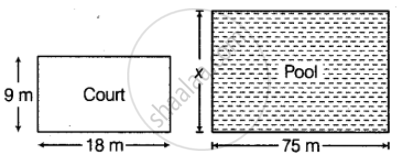# A volleyball court is in a rectangular shape and its dimensions are directly proportional to the dimensions of the swimming pool given below. Find the width of the pool. - Mathematics

Sum

A volleyball court is in a rectangular shape and its dimensions are directly proportional to the dimensions of the swimming pool given below. Find the width of the pool.#### Solution

From the given figures,

Length of volleyball court = 18 m

Breadth of volleyball court = 9 m

Length of pool = 75 m

Let the width of swimming pool = x m

Given, the size of volleyball court and swimming pool are in direct proportion to each other.

∴ 9/18 = x/75

⇒ x = (75 xx 9)/18 = 75/2 = 37.5 m  .....[By corss-multiplication]

Hence, the width of the swimming pool is 37.5 m

Concept: Concept of Direct Proportion
Is there an error in this question or solution?

#### APPEARS IN

NCERT Mathematics Exemplar Class 8
Chapter 10 Direct and Inverse Proportions
Exercise | Q 96 | Page 327